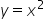Maths-
General
Easy

###Hint:

Integration, as we all know, is the process of determining an area by first dividing it into a number of basic strips and then adding up each one. At this point, we can calculate the area enclosed by a curve and a line connecting a given set of points. Here we have given the curve y=3x and. We have to find the area bounded by the given curve.

#### We are aware that in a planar lamina, the region inhabited by two-dimensional forms is expressed as an area. Calculus requires that you know the difference between two definite integrals of a function in order to calculate the area between two curves. The definite integral of one function, such as f(x), minus the definite integral of other functions, such as g(x), with the lower and upper bounds as a and b, respectively, is used to define the area between the two curves or functions.Here we have given the curve as y=3x and.So now here we can say that using the integration method, the area of the region bounded by the given curves is 4.5. The equation A = ∫ab f(x) dx gives the area under the curve y = f(x) and x-axis. The bounding values for the curve with respect to the x-axis are shown here as a and b.

## Book A Free Demo+91